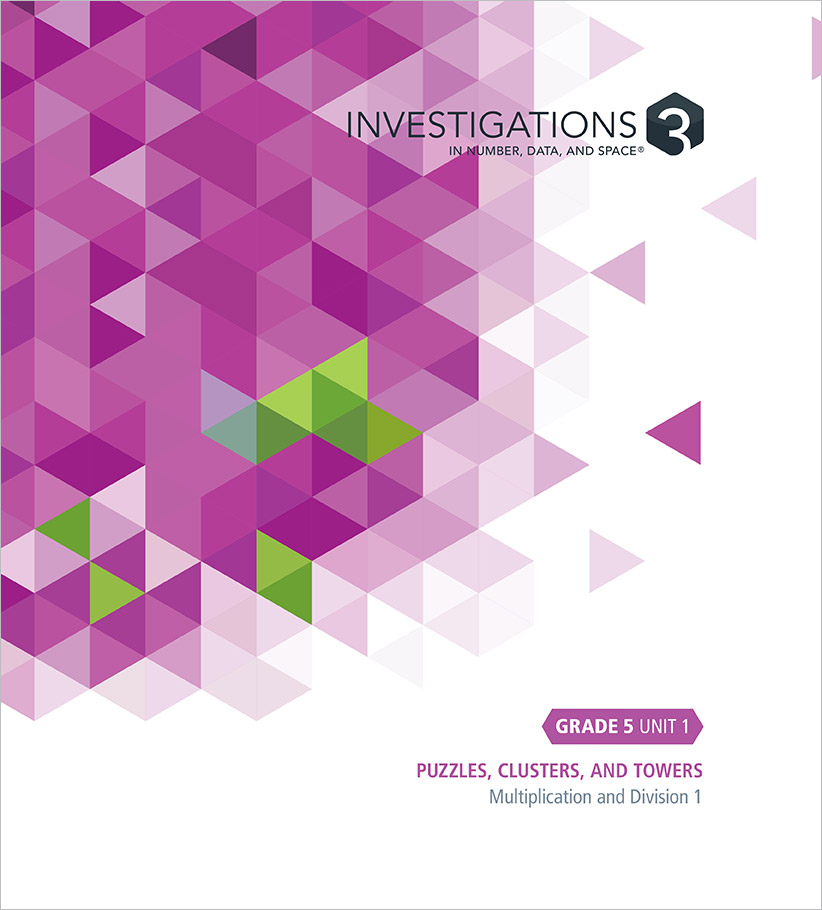Select Page

#### Unit 1: Puzzles, Clusters, and Towers (19 Sessions)

##### Multiplication and Division 1

This unit focuses on the operations of multiplication and division. Students refine their strategies for solving multiplication problems with 2-digit numbers, and use the relationship between multiplication and division to develop and practice strategies for solving division problems. They use order of operations to solve computation problems.

• Investigation 1: Properties of Numbers (5 Sessions)
• Investigation 2: Multiplication Strategies (7 Sessions)
• Investigation 3: Division Strategies (7 Sessions)

#### Unit 2: Prisms and Solids (12 Sessions)

##### 3-D Geometry and Measurement

This unit focuses on the structure and volume of three-dimensional (3-D) shapes, specifically on rectangular prisms and solids composed of rectangular prisms. Students build models and patterns for boxes that hold quantities of cubes and calculate the volume of these boxes, using a cube as a unit of measure. Because volume is additive, students find the volume of solids by decomposing them into rectangular prisms. They use standard units of measure for volume and apply formulas for volume as they determine the volume of a variety of rectangular solids.

• Investigation 1: Finding the Volume of Solids (8 Sessions)
• Investigation 2: Using Standard Cubic Units (4 Sessions)

#### Unit 3: Rectangles, Clocks, and Tracks (19 Sessions)

##### Rational Numbers 1: Addition and Subtraction

This unit focuses on deepening and extending students’ understanding of fractions and equivalent fractions and representing fractions using an area model (rectangles), a rotation model (a clock), and a linear model (number lines). They use these understandings to add and subtract fractions and mixed numbers.

• Investigation 1: Comparing and Ordering Fractions (6 Sessions)
• Investigation 2: Adding and Subtracting Fractions (7 Sessions)
• Investigation 3: Adding and Subtracting Mixed Numbers (6 Sessions)

#### Unit 4: How Many People and Teams? (17 Sessions)

##### Multiplication and Division 2

This unit focuses on the operations of multiplication and division. Students refine their strategies for solving multiplication problems fluently, including using the U.S. standard algorithm. Students continue using the relationship between multiplication and division to efficiently solve division problems with 4-digit dividends and 2-digit divisors.

• Investigation 1: Multiplication Strategies (5 Sessions)
• Investigation 2: Division Strategies and Notation (7 Sessions)
• Investigation 3: Using the Operations (5 Sessions)

#### Unit 5: Temperature, Height, and Growth (14 Sessions)

##### Analyzing Patterns and Rules

This unit focuses on using coordinate graphs, ordered pairs, tables, and symbolic notation to model real world and mathematical situations. Students analyze arithmetic patterns in tables and the shapes of graphs to describe and compare these situations. Students work both with situations that follow patterns, allowing predictions of future values (e.g., how the area of a square varies as the length of a side increases) and situations based on data (e.g., temperature over time).

• Investigation 1: Graphing Temperature and Height (7 Sessions)
• Investigation 2: Analyzing Geometric Patterns (7 Sessions)

#### Unit 6: Between 0 and 1 (17 Sessions)

##### Rational Numbers 2: Addition and Subtraction

This unit focuses on deepening and extending students’ understanding of decimals and the base-10 number system. Students represent decimals on grids and number lines. They use their understanding of decimals to compare, add, and subtract decimals.

• Investigation 1: Representing and Comparing Decimals (8 Sessions)
• Investigation 2: Adding and Subtracting Decimals (9 Sessions)

#### Unit 7: Races, Arrays, and Grids (26 Sessions)

##### Rational Numbers 3: Multiplication and Division

This unit focuses on multiplying and dividing rational numbers, which includes extending students’ understanding of the meaning of those operations and of place value. Students use contexts and representations (fraction bars, arrays, and grids) to solve problems involving multiplication and division of fractions and decimals. Students also apply their understandings of multiplication and division to solve measurement conversion problems.

• Investigation 1: Multiplying and Dividing Fractions (11 Sessions)
• Investigation 2: Fractions as Division (4 Sessions)
• Investigation 3: Multiplying and Dividing Decimals (11 Sessions)

#### Unit 8: Properties of Polygons (10 Sessions)

##### 2-D Geometry and Measurement

This unit focuses on classifying triangles and quadrilaterals based on their properties and on using patterns to describe how the perimeters and areas of rectangles change when the dimensions of the rectangle change. Students examine how categories of polygons are related and how a figure can belong to more than one category. As they build sequences of related rectangles, they analyze numerical relationships and practice adding and multiplying mixed numbers and decimals.

• Investigation 1: Categories and Properties of Polygons (5 Sessions)
• Investigation 2: Finding Perimeter and Area of Related Rectangles (5 Sessions)